A previous article shows that you can use the Intercept parameter to control the ratio of events to nonevents in a simulation of data from a logistic regression model. If you decrease the intercept parameter, the probability of the event decreases; if you increase the intercept parameter, the probability of the event increases.

The probability of Y=1 also depends on the slope parameters (coefficients of the regressor effects) and on the distribution of the explanatory variables. This article shows how to visualize the probability of an event as a function of the regression parameters. I show the visualization for two one-variable logistic models. For the first model, the distribution of the explanatory variable is standard normal. In the second model, the distribution is exponential.

### Visualize the probability as the Intercept varies

In a previous article, I simulated data from a one-variable binary logistic model. I simulated the explanatory variable, X, from a standard normal distribution. The linear predictor is given by η = β0 + β1 X. The probability of the event Y=1 is given by μ = logistic(η) = 1 / (1 + exp(-η)).

In the previous article, I looked at various combinations of Intercept (β0) and Slope (β1) parameters. Now, let's look systematically at how the probability of Y=1 depends on the Intercept for a fixed value of Slope > 0. Most of the following program is repeated and explained in my previous post. For your convenience, it is repeated here. First, the program simulates data (N=1000) for a variable, X, from a standard normal distribution. Then it simulates a binary variable for a series of binary logistic models as the intercept parameter varies on the interval [-3, 3]. (To get better estimates of the probability of Y=1, the program simulates 100 data sets for each value of Intercept.) Lastly, the variable calls PROC FREQ to compute the proportion of simulated events (Y=1), and it plots the proportion versus the Intercept value.

/* simulate X ~ N(0,1) */ data Explanatory; call streaminit(12345); do i = 1 to 1000; x = rand("Normal", 0, 1); /* ~ N(0,1) */ output; end; drop i; run;   /* as Intercept changes, how does P(Y=1) change when Slope=2? */ %let Slope = 2; data SimLogistic; call streaminit(54321); set Explanatory; do Intercept = -3 to 3 by 0.2; do nSim = 1 to 100; /* Optional: param estimate is better for large samples */ eta = Intercept + &Slope*x; /* eta = linear predictor */ mu = logistic(eta); /* mu = Prob(Y=1) */ Y = rand("Bernoulli", mu); /* simulate binary response */ output; end; end; run;   proc sort data=SimLogistic; by Intercept; run; ods select none; proc freq data=SimLogistic; by Intercept; tables Y / nocum; ods output OneWayFreqs=FreqOut; run; ods select all;   title "Percent of Y=1 in Logistic Model"; title2 "Slope=&Slope"; footnote J=L "X ~ N(0,1)"; proc sgplot data=FreqOut(where=(Y=1)); series x=Intercept y=percent; xaxis grid; yaxis grid label="Percent of Y=1"; run;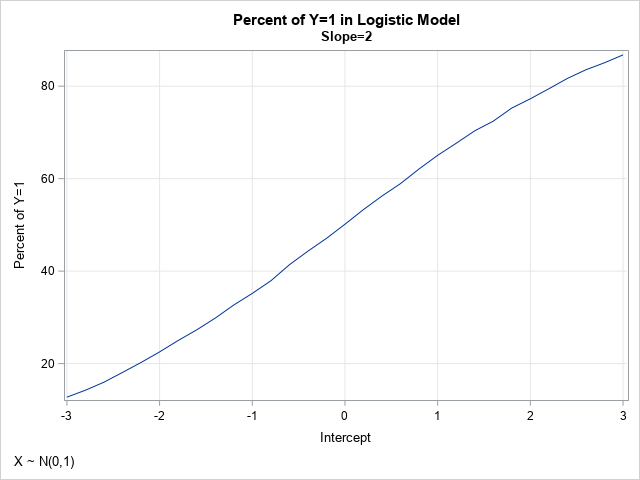In the simulation, the slope parameter is set to Slope=2, and the Intercept parameter varies systematically between -3 and 3. The graph visualizes the probability (as a percentage) that Y=1, given various values for the Intercept parameter. This graph depends on the value of the slope parameter and on the distribution of the data. It also has random variation, due to the call to generate Y as a random Bernoulli variate. For these data, the graph shows the estimated probability of the event as a function of the Intercept parameter. When Intercept = –2, Pr(Y=1) = 22%; when Intercept = 0, Pr(Y=1) = 50%; and when Intercept = 2, Pr(Y=1) = 77%. The statement that Pr(Y=1) = 50% when Intercept=0 should be approximately true when the data is from a symmetric distribution with zero mean.

### Vary the slope and intercept together

In the previous section, the slope parameter is fixed at Slope=2. What if we allow the slope to vary over a range of values? We can restrict our attention to positive slopes because logistic(η) = 1 – logistic(-η). The following program varies the Intercept parameter in the range [-3,3] and the Slope parameter in the range [0, 4].

/* now do two-parameter simulation study where slope and intercept are varied */ data SimLogistic2; call streaminit(54321); set Explanatory; do Slope = 0 to 4 by 0.2; do Intercept = -3 to 3 by 0.2; do nSim = 1 to 50; /* optional: the parameter estimate are better for larger samples */ eta = Intercept + Slope*x; /* eta = linear predictor */ mu = logistic(eta); /* transform by inverse logit */ Y = rand("Bernoulli", mu); /* simulate binary response */ output; end; end; end; run;   /* Monte Carlo estimate of Pr(Y=1) for each (Int,Slope) pair */ proc sort data=SimLogistic2; by Intercept Slope; run; ods select none; proc freq data=SimLogistic2; by Intercept Slope; tables Y / nocum; ods output OneWayFreqs=FreqOut2; run; ods select all;

The output data set (FreqOut2) contains Monte Carlo estimates of the probability that Y=1 for each pair of (Intercept, Slope) parameters, given the distribution of the explanatory variable. You can use a contour plot to visualize the probability of the event for each combination of slope and intercept:

/* Create template for a contour plot https://blogs.sas.com/content/iml/2012/07/02/create-a-contour-plot-in-sas.html */ proc template; define statgraph ContourPlotParm; dynamic _X _Y _Z _TITLE _FOOTNOTE; begingraph; entrytitle _TITLE; entryfootnote halign=left _FOOTNOTE; layout overlay; contourplotparm x=_X y=_Y z=_Z / contourtype=fill nhint=12 colormodel=twocolorramp name="Contour"; continuouslegend "Contour" / title=_Z; endlayout; endgraph; end; run; /* render the Monte Carlo estimates as a contour plot */ proc sgrender data=Freqout2 template=ContourPlotParm; where Y=1; dynamic _TITLE="Percent of Y=1 in a One-Variable Logistic Model" _FOOTNOTE="X ~ N(0, 1)" _X="Intercept" _Y="Slope" _Z="Percent"; run;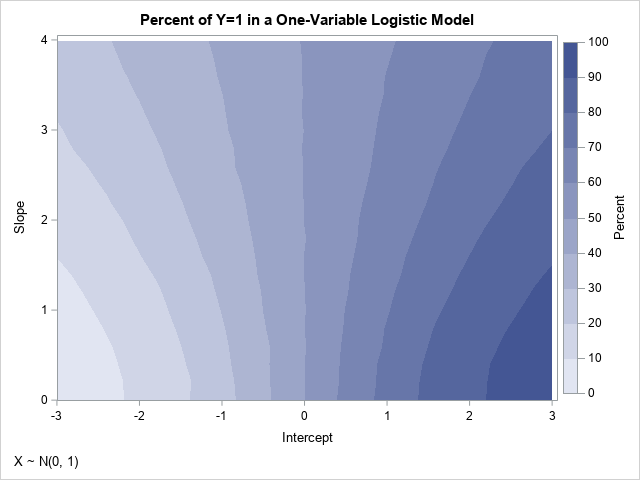As mentioned earlier, because X is approximately symmetric, the contours of the graph have reflective symmetry. Notice that the probability that Y=1 is 50% whenever Intercept=0 for these data. Furthermore, if the points (β0, β1) are on the contour for Pr(Y=1)=α, then the contour for Pr(Y=1)=1-α contains points close to (-β0, β1). The previous line plot is equivalent to slicing the contour plot along the horizontal line Slope=2.

You can use a graph like this to simulate data that have a specified probability of Y=1. For example, if you want approximately 70% of the cases to be Y=1, you can choose any pair of (Intercept, Slope) values along that contour, such as (0.8, 0), (1, 1), (2, 3.4), and (2.4, 4). If you want to see all parameter values for which Pr(Y=1) is close to a desired value, you can use the WHERE statement in PROC PRINT. For example, the following call to PROC PRINT displays all parameter values for which the Pr(Y=1) is approximately 0.7 or 70%:

%let Target = 70; proc print data=FreqOut2; where Y=1 and %sysevalf(&Target-1) <= Percent <= %sysevalf(&Target+1); var Intercept Slope Y Percent; run;

### Probabilities for nonsymmetric data

The symmetries in the previous graphs are a consequence of the symmetry in the data for the explanatory variable. To demonstrate how the graphs change for a nonsymmetric data distribution, you can run the same simulation study, but use data that are exponentially distributed. To eliminate possible effects due to a different mean and variance in the data, the following program standardizes the explanatory variable so that it has zero mean and unit variance.

data Expo; call streaminit(12345); do i = 1 to 1000; x = rand("Expon", 1.5); /* ~ Exp(1.5) */ output; end; drop i; run;   proc stdize data=Expo method=Std out=Explanatory; var x; run;

If you rerun the simulation study by using the new distribution of the explanatory variable, you obtain the following contour plot of the probability as a function of the Intercept and Slope parameters: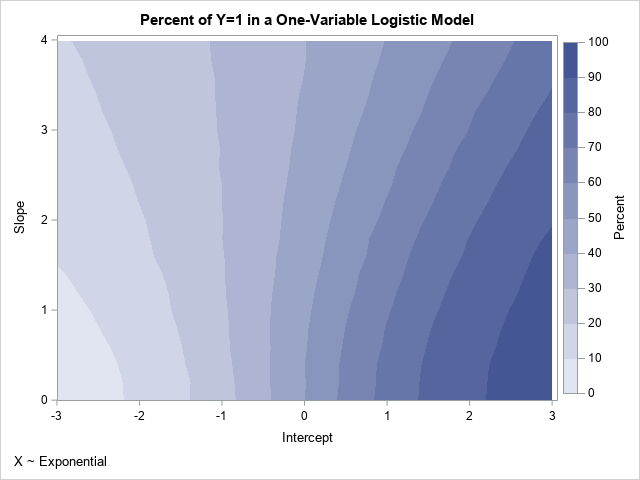If you compare this new contour plot to the previous one, you will see that they are very similar for small values of the slope parameter. However, they are different for larger values such as Slope=2. The contours in the new plot do not show reflective symmetry about the vertical line Intercept=0. Pairs of parameters for which Pr(Y=1) is approximately 0.7 include (0.8, 0) and (1, 1), which are the same as for the previous plot, and (2.2, 3.4), and (2.6, 4), which are different from the previous example.

### Summary

This article presents a Monte Carlo simulation study in SAS to compute and visualize how the Intercept and Slope parameters of a one-variable logistic model affect the probability of the event. A previous article notes that decreasing the Intercept decreases the probability. This article shows that the probability depends on both the Intercept and Slope parameters. Furthermore, the probability depends on the distribution of the explanatory variables. You can use the results of the simulation to control the proportion of events to nonevents in the simulated data.

The ideas in this article generalize to logistic regression models that contain multiple explanatory variables. For multivariate models, the effect of the Intercept parameter is similar. However, the effect of the slope parameters is more complicated, especially when the variables are correlated with each other.

This article shows that you can use the intercept parameter to control the probability of the event in a simulation study that involves a binary logistic regression model. For simplicity, I will simulate data from a logistic regression model that involves only one explanatory variable, but the main idea applies to multivariate regression models. I also show mathematically why the intercept parameter controls the probability of the event.

### The problem: Unbalance events when simulating regression data

Usually, simulation studies are based on real data. From a set of data, you run a statistical model that estimates parameters. Assuming the model fits the data, you can simulate new data by using the estimates as if they were the true parameters for the model. When you start with real data, you obtain simulated data that often have similar statistical properties.

However, sometimes you might want to simulate data for an example, and you don't have any real data to model. This is often the case on online discussion forums such as the SAS Support Community. Often, a user posts a question but cannot post the data because it is proprietary. In that case, I often simulate data to demonstrate how to use SAS to answer the question. The simulation is usually straightforward for univariate data or for data from an ordinary least squares regression model. However, the simulation can become complicated for generalized linear regression models and nonlinear models. Why? Because not every choice of parameters leads to a reasonable data set. For example, if you choose parameters "out of thin air" in an arbitrary manner, you might end up with a model for which the probability of the event (Pr(Y=1)) is close to 1 for all values of the explanatory variables. This model is not very useful.

It turns out that you can sometimes "fix" your model by decreasing the value of the intercept parameter in the model. This reduces the value of Pr(Y=1), which can lead to a more equitable and balanced distribution of the response values.

### Simulate and visualize a one-variable binary logistic regression model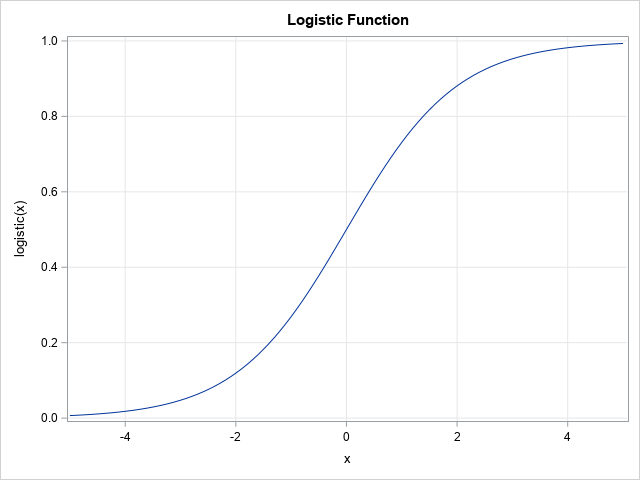Let's construct an example. For a general discussion and a multivariate example, see the article, "Simulating data for a logistic regression model." For this article, let's keep it simple and use a one-variable model. Let X be an independent variable that is distributed as a standardized normal variate: X ~ N(0,1). Then choose an intercept parameter, β0, and a slope parameter, β1, and form the linear predictor η = β0 + β1 X. A binary logistic model uses a logistic transformation to transform the linear predictor to a probability: μ = logistic(η), where logistic(η) = 1 / (1 + exp(-η)). The graph of the S-shaped logistic function is shown to the right. You can then simulate a response as a Bernoulli random variable Y ~ Bern(μ).

In SAS, the following DATA step simulates data from a standard normal variable:

data Explanatory; call streaminit(12345); do i = 1 to 1000; x = rand("Normal", 0, 1); /* ~ N(0,1) */ output; end; drop i; run;

Regardless of the distribution of X, the following DATA step simulates data from a logistic model that has a specified set of values for the regression parameters. I embed the program in a SAS macro so that I can run several models with different values of the Intercept and Slope parameters. The macro also runs PROC FREQ to determine the relative proportions of the binary response Y=0 and Y=1. Lastly, the macro calls PROC SGPLOT to display a graph that shows the logistic regression model and the "observed" responses as a function of the linear predictor values,

/* Simulate data from a one-variable logistic model. X ~ N(0,1) and Y ~ Bern(eta) where eta = logistic(Intercept + Slope*X). Display frequency table for Y and a graph of Y and Prob(Y=1) vs eta */ %macro SimLogistic(Intercept, Slope); title "Logistic Model for Simulated Data"; title2 "Intercept=&Intercept; Slope=&Slope"; data Sim1; call streaminit(54321); set Explanatory; eta = &Intercept + &Slope*x; /* eta = linear predictor */ mu = logistic(eta); /* mu = Prob(Y=1) */ Y = rand("Bernoulli", mu); /* simulate binary response */ run;   proc freq data=Sim1; tables Y / nocum; run; proc sort data=Sim1; by eta; run;   proc sgplot data=Sim1; label Y="Observed" mu="P(Y=1)" eta="Linear Predictor"; scatter x=eta y=Y / transparency=0.8 markerattrs=(symbol=CircleFilled); series x=eta y=mu; xaxis grid values = (-16 to 16) valueshint; yaxis grid label="Probability"; keylegend / location=inside position=E opaque; run; %mend;

### How does the probability of the event depend on the intercept?

Let's run the program. The following call uses an arbitrary choice of values for the regression parameters: Intercept=5 and Slope=2:

/* call the macro with Intercept=5 and Slope=2 */ %SimLogistic(5, 2);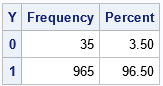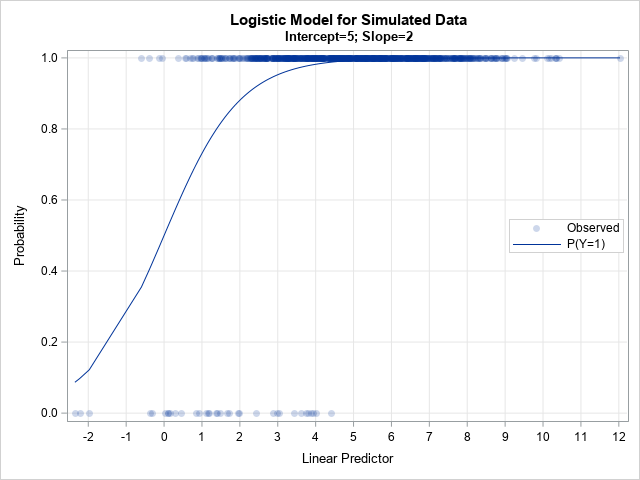The output shows what can happen if you aren't careful when choosing the regression parameters. For this simulation, 96.5% of the response values are Y=1 and only a few are Y=0. This could be a satisfactory model for a rare event, but not for situations in which the ratio of events to nonevents is more balanced, such as 75-25, 60-40, or 50-50 ratios.

The graph shows the problem: the linear predictor (in matrix form, η = X*β) is in the range [-2, 12], and about 95% of the linear prediction are greater than 1.5. Because logistic(1.5) > 0.8, the probability of Y=1 is very high for most observations.

Since you have complete control of the parameters in the simulation, you can adjust the parameters. If you shift η to the left, the corresponding probabilities will decrease. For example, if you subtract 4 from η, then the range of η becomes [-6, 8], which should provide a more balanced distribution of the response category. The following statement implements this choice:

/* call the macro with Intercept=1 and Slope=2 */ %SimLogistic(1, 2);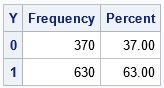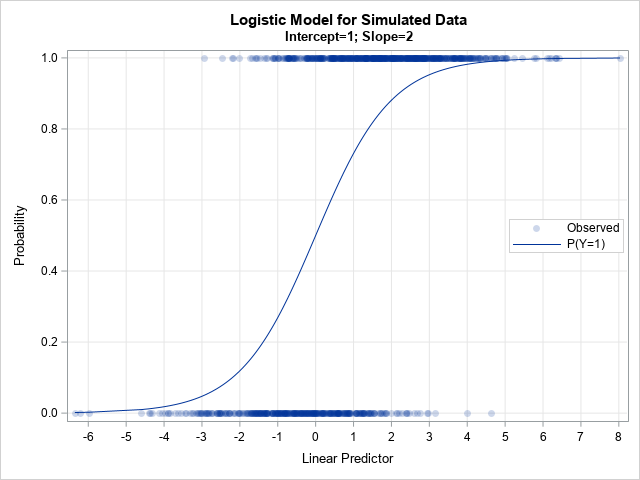The output shows the results of the simulation after decreasing the intercept parameter. When the Intercept=1 (instead of 5), the proportion of simulated responses is more balanced. In this case, 63% of the responses are Y=1 and 37% are Y=0. If you want to further decrease the proportion of Y=1, you can decrease the Intercept parameter even more. For example, if you run %SimLogistic(-1, 2), then only 35.9% of the simulated responses are Y=1.

### The mathematics

I have demonstrated that decreasing η (the linear predictor) also decreases μ (the probability of Y=1). This is really a simple mathematical consequence of the formula for the logistic function. Suppose that η1 < η2. Then -η1 > -η2
⇒    1 + exp(-η1) > 1 + exp(-η2) because EXP is a monotonic increasing function
⇒    1 / (1 + exp(-η1)) < 1 / (1 + exp(-η2)) because the reciprocal function is monotonic decreasing
⇒    μ1 < μ2 by definition.
Therefore, decreasing η results in decreasing μ For some reason, visualizing the simulation makes more of an impact than the math.

### Summary

This article shows that by adjusting the value of the Intercept parameter in a simulation study, you can adjust the ratio of events to nonevents. Decrease the intercept parameter to decrease the number of events; increase the intercept parameter to increase the number of events. I demonstrated this fact for a one-variable model, but the main ideas apply to multivariate models for which the linear predictor (η) is a linear combination of multiple variables.

The probability of Y=1 depends continuously on the regression parameters because the linear predictor and the logistic function are both continuous. In my next article, I show how to visualize the probability of an event as a function of the regression parameters.

A probabilistic card trick is a trick that succeeds with high probability and does not require any skill from the person performing the trick. I have seen a certain trick mentioned several times on social media. I call it "ladders" or the "ladders game" because it reminds me of the childhood board game Chutes and Ladders (previously known as Snakes and Ladders in India and the UK). In Chutes and Ladders, some squares on the board tell the player to move forward (go up a ladder) or to move backward (go down a chute). In the "ladders" game, you only move forward.

I have been told that Martin Gardner wrote about this game. He referred to mathematical card tricks as those that "do not require prestidigitation," or sleight of hand.

The rules of the game and a Python simulation are presented in a blog post by Bob Carpenter, who saw the trick performed at a children's museum. The performer shuffles an ordinary deck of 52 playing cards and places them face up in a row on a table. The performer then describes the game of ladders:

• A player starts by pointing to any card in the first five positions.
• The value of the card indicates the number of cards to move forward. If the card is 2, 3, ..., 9, you move forward that many cards. If the card is a 10, jack, queen, king, or ace, you move forward one space.
• From the new position, apply the rule again. Repeat this process until you cannot advance any more.

The performer selects two volunteers from the audience and tells them each to point randomly to any card from among the first five cards. Each player does so. The performer then announces that both players will end up on a certain "magical" card and he tells everyone the name of the magical card. The players then follow the game rules. Amazingly, each person's path terminates on the magical card that was previously announced. The trick works about 98.2% of the time.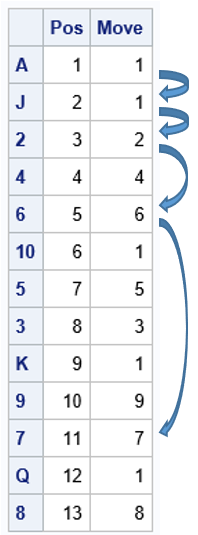To illustrate the ladders game, first consider a simpler situation of a deck that contains only 13 cards, one for each card value (for example, use only spades). One random shuffle of the 13 cards is shown to the right. For this arrangement of cards, the performer announces that both volunteers will end up on the 7 card (the magical card for this shuffle), which is the 11th card in the row of cards. Suppose the first volunteer points to the ace in the first position as her starting card. The rules of the game dictate the following sequence of moves:

• From the A, the player moves forward one card and lands on the J.
• From the J, the player moves forward one card and lands on the 2.
• From the 2, the player moves forward two cards and lands on the 6.
• From the 6, the player moves forward six cards and lands on the 7. The player cannot move forward seven spaces, so the 7 is her final card, as predicted in advance.

Thus, the path for the first player is the sequence of positions (1, 2, 3, 5, 11).

What happens for the other player? Well, if the second volunteer chooses his initial card at positions 2, 3, or 5, then his path will instantly coincide with the path of the first player, so his path, too, terminates at the 7 card in the 11th position. If the second volunteer chooses his initial card at position 4, then his path hits positions 4, 8, and 11. This path also terminates at the 7 card in the 11th position. Thus, for this set of cards and this shuffle, all initial positions follow a path that eventually lands on the 7.

How does the performer know the name of the final magical card? As he deals, he secretly counts the path determined by the first card in the deck.

### Simulate the ladders game in SAS

You can use Monte Carlo simulation to estimate the probability that the ladders card trick "works." To keep it simple, let's say the trick "works" when the paths of the two players converge. That is, the players initially start on two random positions (1-5), but they end up on the same final card after they each follow the path dictated by their initial position. The simulation for the ladders card trick has the following major steps:

1. Set up the card deck. For each card, assign the number of steps to move forward.
2. Create a function that computes the final position, given the initial position and the sequence of moves determined by the shuffled deck.
3. Run the Monte Carlo simulation. This consists of several sub-steps:
• Shuffle the deck (that is, shuffle the array of moves).
• Use sampling without replacement to choose two initial positions.
• Compute the final card for each initial position.
• Record whether the two paths converged.
4. Estimate the probability of the trick working as the proportion of times that the paths converged in the simulation.

As is often the case, this simulation is easiest to perform in SAS by using the SAS/IML language. You can use the RANPERM function to shuffle the deck. You can use the SAMPLE function to sample without replacement from the values 1-5. You can write a function that computes the final position based on the initial position. The following program is one way to implement a Monte Carlo simulation for the ladders card trick:

/* The Ladders card trick: https://statmodeling.stat.columbia.edu/2022/10/07/mira-magic-a-probabilistic-card-trick/ Lay out a shuffled deck of cards. Choose one of the first five cards as a starting point. Move down the deck as follows: If the current card is 2-9, move that many cards forward. For other cards, move one space forward. */ proc iml; /* 1. set up the card deck and assign values. Note: We don't actually need the deck, only the instructions about how to move. */ values = {A,'2','3','4','5','6','7','8','9','10', J, Q, K}; advance= {1, 2, 3, 4, 5, 6, 7, 8, 9, 1, 1, 1, 1}; deck = repeat(values, 4); /* full deck of 52 cards */ moves = repeat(advance, 4); /* corresponding moves */ nCards = nrow(moves);   /* 2. Find the final position, given the first position and the vector of moves for the current shuffled deck. startPos : a scalar value 1-5 Move : a vector of values. If your current card is in position i, the next card is position (i + Move[i]) */ start EndPos(startPos, Move); Pos = startPos; do while(Pos + Move[Pos] < nrow(Move)); Pos = Pos + Move[Pos]; end; return( Pos ); finish;   /* 3. Run the Monte Carlo simulation: A. Shuffle the deck (vector of moves) B. Use sampling w/o replacement to choose two initial positions C. Compute the final card for each initial position D. Record whether the two paths converged */ call randseed(1234); NSim = 1E5; match = j(NSim,1); do j = 1 to nSim; k = ranperm(nCards); /* shuffle the deck */ Move = moves[k]; /* choose two different starting positions in 1-5 */ startPos = sample(1:5, 2, "NoReplace"); EndPos1 = EndPos(startPos, Move); EndPos2 = EndPos(startPos, Move); match[j] = (EndPos1=EndPos2); end;   /* 4. Estimate probability as the proportion of matches (1s) in a binary vector. Optional: Compute a Wald CL for the proportion */ ProbMatch = mean(match); StdErr = sqrt( ProbMatch*(1-ProbMatch)/ NSim ); CL = ProbMatch + {-1 1}*1.96*StdErr; print NSim[F=comma9.] ProbMatch StdErr CL[c={'LCL' 'UCL'}];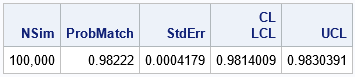The simulation shows that the two players end up on the same card more than 98% of the time.

### Summary

This article describes a probabilistic card trick, which I call the game of ladders. Two players choose random cards as an initial condition, then follow the rules of the game. For about 98.2% of the deals and initial positions, the paths of the two players will converge to the same card. As the performer is laying out the card, he can predict in advance that the paths will converge and, with high probability, announce the name of the final card.

Bob Carpenter's blog post mentions the connection between this card trick and Markov chains. He also presents several variations, such as using more cards in the deck.

The post Ladders: A probabilistic card trick appeared first on The DO Loop.

A SAS programmer was trying to simulate poker hands. He was having difficulty because the sampling scheme for simulating card games requires that you sample without replacement for each hand. In statistics, this is called "simple random sampling."

If done properly, it is straightforward to simulate poker hands in SAS. This article shows how to generate a standard deck of 52 cards. It then shows how to generate many card hands for a game that involves several players. This can be done in two ways: The SAMPLE function in the SAS/IML language and PROC SURVEYSELECT in SAS/STAT software.

### Encode a deck of cards

The first step is to generate a standard deck of 52 playing cards. There are many ways to do this, but the following DATA step iterates over 13 values (A, 2, 3, ..., Q, K) and over four suits (clubs, diamonds, hearts, and spades). The step creates a data set that has 52 cards. To make the output more compact, I concatenate the value of the card with the first letter of the suit to obtain a two-character string for each card. For example, "5C" is the "five of clubs" and "AS" is the "ace of spades." So that each card can be represented by using two characters, I use "T" instead of "10." For example, "TH" is the "ten of hearts." The

data Cards; length Value $2 Suit$8 Card $3; array Values$ _temporary_ ('A','2','3','4','5','6','7','8','9','T','J','Q','K'); array Suits $_temporary_ ('Clubs', 'Diamonds', 'Hearts', 'Spades'); do v = 1 to 13; do s = 1 to 4; Value = Values[v]; Suit = Suits[s]; Card = cats(Value,substr(Suit,1,1)); output; end; end; run; proc print data=Cards(obs=8); var v s Value Suit Card; run; TABLE The cards are generated in order. The output shows the first eight cards in the deck, which are the aces and twos. For some types of poker hands (such as determining pairs and straights), the V variable can be useful, and for other analyses (such as determining flushes), the S variable can be useful. ### Deal cards by using SAS/IML When humans deal cards, we use a two-step method: shuffle the deck and then deal the top cards to players in a round-robin fashion. A computer simulation can simluate dealing cards by using a one-step method: deal random cards (without replacement) to every player. SAS provides built-in sampling methods to make this process easy to program. In SAS/IML, the default sampling method for the SAMPLE function is sampling without replacement. If there are P players and each player is to receive C cards, then a deal consists of P*C cards, drawn without replacement from the deck. The deck does not need to be shuffled because the SAMPLE function outputs the cards in random order. Because the cards are in random order, it does not matter how you assign the cards to the players. The following SAS/IML program uses P=3 players and generates C=5 cards for each player. The program simulates one deal by assigning the first C cards to the first player, the second C cards to the second player, and so on: %let nCards = 5; /* cards per player */ %let nPlayers = 3; /* number of players */ %let nDeals = 10; /* number of deals to simulate */ /* simulate dealing many card hands in SAS/IML */ proc iml; call randseed(1234); /* set random number seed */ use Cards; read all var "Card"; close; /* read the deck of cards into a vector */ nCardsPerDeal = &nCards * &nPlayers; /* each row is a complete deal */ D = sample(Card, nCardsPerDeal, "WOR" ); /* sample without replacement from the deck */ Deal = shape(D, &nPlayers, &nCards); /* reshape into nPlayers x nCards matrix */ /* print one deal */ cardNames = "C1":"C&nCards"; playerNames = "P1":"P&nPlayers"; print Deal[c=cardNames r=playerNames]; TABLE For this deal, the first player has two tens, two fives, and a king. The second player has a four, a five, a nine, an ace, and a two. You could sort the cards in each row, but I have left each player's cards unsorted so that you can see the random nature of the assignment. ### Simulate multiple deals The previous program simulates one deal. What if you want to simulate multiple deals? One way is to loop over the number of deals, but there is a more efficient method. The second argument to the SAMPLE function can be a two-element vector. The first element specifies the number of cards for each deal, and the second element specifies the number of deals. Thus, for example, if you want to simulate 10 deals, you can use the following statement to generate a matrix that has 10 rows. Each row is a sample (without replacement) from a 52-card deck: /* use one call to simulate many deals */ D = sample(Card, nCardsPerDeal//&nDeals, "WOR" ); /* simulate many deals */ /* Ex: The 8th row contains the 8th deal. Display it. */ Deal8 = shape(D[8,], &nPlayers, &nCards); print Deal8[c=cardNames r=playerNames]; TABLE To demonstrate that each row is a deal, I have extracted, reshaped, and displayed the eighth row. The eighth deal is unusual because each player is dealt a "good" hand. The first player has a pair of eights, the second player has two pairs (threes and sixes), and the third player has a pair of sevens. If you want to print (or analyze) the 10 deals, you can loop over the rows, as follows: do i = 1 to &nDeals; Deal = shape(D[i,], &nPlayers, &nCards); /* analyze or print the i_th deal */ print i, Deal[c=cardNames r=playerNames]; end; ### Deal random hands by using PROC SURVEYSELECT You can also simulate card deals by using the SURVEYSELECT procedure. You should use the following options: • Use the METHOD=SRS option to specify sampling without replacement for each deal. To obtain the cards in random order, use the OUTRANDOM option. • Use the N= option to specify the number of cards in each deal. • Use the REPS= option to specify the total number of deals. An example is shown in the following call to PROC SURVEYSELECT: /* similar approach for PROC SURVEYSELECT */ proc surveyselect data=Cards seed=123 NOPRINT method=SRS /* sample without replacement */ outrandom /* randomize the order of the cards */ N=%eval(&nCards * &nPlayers) /* num cards per deal */ reps=&nDeals /* total number of deals */ out=AllCards; run; The output data set (AllCards) contains a variable named Replicate, which identifies the cards in each deal. Because the cards for each deal are in a random order, you can arbitrarily assign the cards to players. You can use a round-robin method or assign the first C cards to the first player, the next C cards to the second player, and so on. This is done by using the following DATA step: /* assign the cards to players */ data AllDeals; set AllCards; by Replicate; if first.Replicate then do; Cnt = 0; end; Player = 1 + floor(Cnt/&nCards); Cnt + 1; drop Cnt; run; OUTPUT The simulated data are in long form. For some analyses, it might be more convenient to convert it into wide form. You can do this by using the DATA step or by using PROC TRANSPOSE, as below: /* OPTIONAL: Convert from long form to wide form */ proc transpose data=AllDeals out=Deals(rename=(col1-col&nCards=C1-C&nCards) drop=_NAME_); var Card; by Replicate Player; run; proc print data=Deals; where Replicate=8; var Replicate Player C:; run; OUTPUT To demonstrate the results, I display the result of the eighth deal (Replicate=8). For this deal, the first player has several face cards but no pairs, the second player has a pair of threes, and the third player has a pair of fours. ### Summary SAS provides many tools for simulation studies. For simulations of card games, this article shows how to create a data set that represents a standard deck of 52 playing cards. From the cards, you can use the SAMPLE function in SAS/IML software or the SURVEYSELECT procedure to simulate many deals, where each deal is a sample without replacement from the deck. For each deal, you can assign the cards to the players in a systematic manner. The post Simulate poker hands in SAS appeared first on The DO Loop. I recently blogged about how to compute the area of the convex hull of a set of planar points. This article discusses the expected value of the area of the convex hull for n random uniform points in the unit square. The article introduces an exact formula (due to Buchta, 1984) and uses Monte Carlo simulation to approximate the sampling distribution of the area of a convex hull. A simulation like this is useful when testing data against the null hypothesis that the points are distributed uniformly at random. ### The expected value of a convex hull Assume that you sample n points uniformly at random in the unit square, n ≥ 3. What is the expected area of the convex hull that contains the points? Christian Buchta ("Zufallspolygone in konvexen Vielecken," 1984) proved a formula for the expected value of the area of n random points in the unit square. (The unit square is sufficient: For any other rectangle, simply multiply the results for the unit square by the area of the rectangle.) Unfortunately, Buchta's result is published in German and is behind a paywall, but the formula is given as an answer to a question on MathOverflow by 'user9072'. Let $s = \sum_{k=1}^{n+1} \frac{1}{k} (1 - \frac{1}{2^k})$. Then the expected area of the convex hull of n points in [0,1] x [0,1] is $E[A(n)] = 1 -\frac{8}{3(n+1)} \bigl[ s - \frac{1}{(n+1)2^{n+1}} \bigr]$ The following SAS DATA step evaluates Buchta's formula for random samples of n ≤ 100. A few values are printed. You can use PROC SGPLOT to graph the expected area against n: /* Expected area of the convex hull of a random sample of size n in the unit square. Result by Buchta (1984, in German), as reported by https://mathoverflow.net/questions/93099/area-enclosed-by-the-convex-hull-of-a-set-of-random-points */ data EArea; do n = 3 to 100; s = 0; do k = 1 to n+1; s + (1 - 1/2**k) / k; end; EArea = 1 - 8/3 /(n+1) * (s - 2**(-n-1) / (n+1)); output; end; keep n EArea; run; proc print data=EArea noobs; where n in (3,4,6,8,10,12,13) or mod(n,10)=0; run; title "Expected Area of Convex Hull"; proc sgplot data=EArea; series x=n y=EArea; xaxis grid label="Number of Random Points in Unit Square"; yaxis grid label="Expected Area" min=0 max=1; run;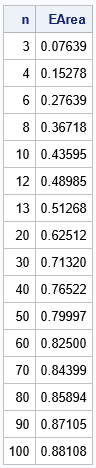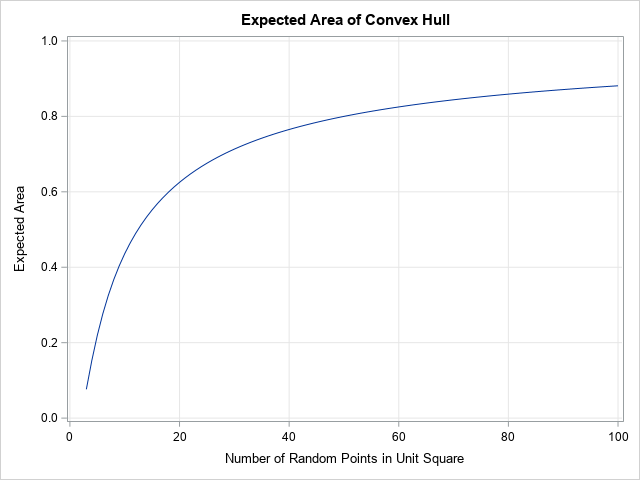The computation shows that when n is small, the expected area, E[A(n)], is very small. The first few values are E[A(3)]=11/144, E[A(4)]=11/72, E[A(5)] = 79/360, and E[A(6)] = 199/720. For small n, the expected area increases quickly as n increases. When n = 12, the expected area is about 0.5 (half the area of the bounding square). As n gets larger, the expected area asymptotically approaches 1 very slowly. For n = 50, the expected area is 0.8. For n = 100, the expected area is 0.88. ### The distribution of the expected area of a convex hull Buchta's result gives the expected value, but the expected value is only one number. You can use Monte Carlo simulation to compute and visualize the distribution of the area statistics for random samples. The distribution enables you to estimate other quantities, such as the median area and an interval that predicts the range of the areas for 95% of random samples. The following SAS/IML program performs a Monte Carlo simulation to approximate the sampling distribution of the area of the convex hull of a random sample in the unit square of size n = 4. The program does the following: 1. Load the PolyArea function, which was defined in a previous article that shows how to compute the area of a convex hull. Or you can copy/paste from that article. 2. Define a function (CHArea) that computes the convex hull of a set of points and returns the area. 3. Let B be the number of Monte Carlo samples, such as B = 10,000. Use the RANDFUN function to generate B*n random points in the unit square. This is an efficient way to generate B samples of size n. 4. Loop over the samples. For each sample, extract the points in the sample and call the CHArea function to compute the area. 5. The Monte Carlo simulation computes B sample areas. The distribution of the areas is the Monte Carlo approximation of the sampling distribution. You can compute descriptive statistics such as mean, median, and percentiles to characterize the shape of the distribution. proc iml; /* load the PolyArea function or copy it from https://blogs.sas.com/content/iml/2022/11/02/area-perimeter-convex-hull.html */ load module=(PolyArea); /* compute the area of the convex hull of n pts in 2-D */ start CHArea(Pts); indices = cvexhull(Pts); /* compute convex hull of the N points */ hullIdx = indices[loc(indices>0)]; /* the positive indices are on the CH */ P = Pts[hullIdx, ]; /* extract rows to form CH polygon */ return PolyArea(P); /* return the area */ finish; call randseed(1234); NSim = 1E4; /* number of Monte Carlo samples */ n = 4; X = randfun((NSim*n)//2, "Uniform"); /* matrix of uniform variates in [0,1]x[0,1] */ Area = j(NSim, 1, .); /* allocate array for areas */ do i = 1 to NSim; beginIdx = (i-1)*n + 1; /* first obs for this sample */ ptIdx = beginIdx:(beginIdx+n-1); /* range of obs for this sample */ Pts = X[ptIdx, ]; /* use these n random points */ Area[i] = CHArea(Pts); /* find the area */ end; meanArea = mean(Area); medianArea = median(Area); call qntl(CL95, Area, {0.025 0.975}); print n meanArea medianArea (CL95)[c={LCL UCL}];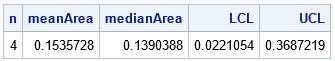For samples of size n = 4, the computation shows that the Monte Carlo estimate of the expected area is 0.154, which is very close to the expected value (0.153). In addition, the Monte Carlo simulation enables you to estimate other quantities, such as the median area (0.139) and a 95% interval estimate for the predicted area ([0.022, 0.369]). You can visualize the distribution of the areas by using a histogram. The following SAS/IML statements create a histogram and overlay a vertical reference line at the expected value. title "Distribution of Areas of Convex Hull"; title2 "N=4; NSim = 10,000"; refStmt = "refline 0.15278 / axis=x lineattrs=(color=red) label='E(Area)';"; call histogram(Area) other=refStmt;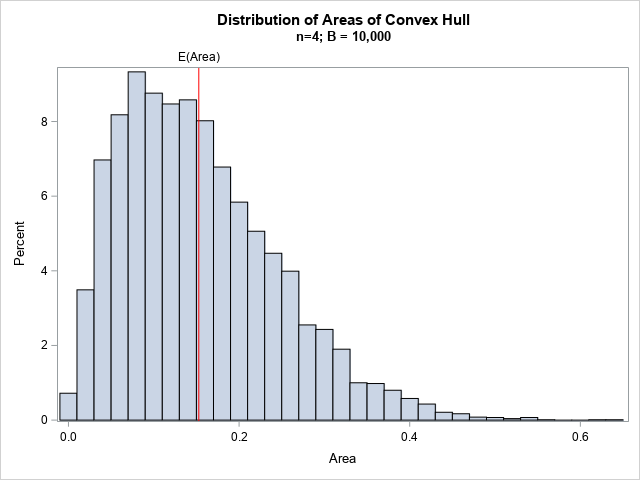The histogram shows that the distribution of areas is skewed to the right for n = 4. For this distribution, a better measure of centrality might be the median, which is smaller than the expected value (the mean). Or you can describe the distribution by reporting a percentile range such as the inter-quartile range or the range for 95% of the areas. ### Graphs of the distribution of the area of a convex hull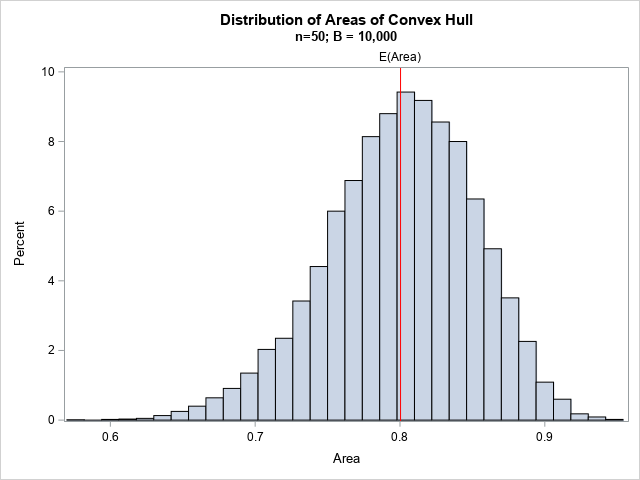You can repeat the previous Monte Carlo simulation for other values of n. For example, if you use n = 50, you get the histogram shown to the right. The distribution for n = 50 is skewed to the left. A prediction interval for 95% of areas is [0.69, 0.89]. Whether or not you know about Buchta's theoretical result, you can use Monte Carlo simulation to estimate the expected value for any sample size. The following SAS/IML program simulates 10,000 random samples of size n for n in the range [3, 100]. For each simulation, the program writes the estimate of the mean and a prediction interval to a SAS data set. You can use the BAND statement in PROC SGPLOT to overlay the interval estimates and the estimate of the expected value for each value of n: /* repeat Monte Carlo simulation for many values of N. Write results to a data set and visualize */ create ConvexHull var {'n' MeanArea LCL UCL}; numPts = 3:100; NSim = 1E4; /* number of Monte Carlo samples */ do j = 1 to ncol(numPts); /* number of random pts for each convex hull */ N = numPts[j]; X = randfun((NSim*N)//2, "Uniform"); /* matrix of uniform variates in [0,1]x[0,1] */ Area = j(NSim, 1, .); /* allocate array for areas */ LCL = j(NSim, 1, .); /* allocate arrays for 95% lower/upper CL */ UCL = j(NSim, 1, .); do i = 1 to NSim; beginIdx = (i-1)*N + 1; /* first obs for this sample */ ptIdx = beginIdx:(beginIdx+N-1); /* range of obs for this sample */ Pts = X[ptIdx, ]; /* use these N random points */ Area[i] = CHArea(Pts); end; meanArea = mean(Area); call qntl(CL95, Area, {0.025 0.975}); LCL = CL95; UCL = CL95; append; end; close ConvexHull; QUIT; title "Monte Carlo Estimate of Expected Area of Convex Hull"; proc sgplot data=ConvexHull noautolegend; band x=n lower=LCL upper=UCL / legendlabel="95% Prediction"; series x=n y=meanArea / legendlabel="Expected Area"; keylegend / location=inside position=E opaque across=1; xaxis grid label="Number of Random Points in Unit Square"; yaxis grid label="Expected Area" min=0 max=1; run;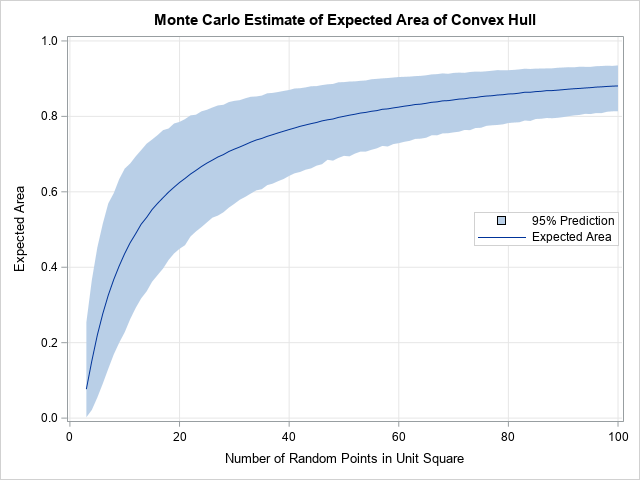From this graph, you can determine the expected value and a 95% prediction range for any sample size, 3 ≤ n ≤ 100. Notice that the solid line is the Monte Carlo estimate of the expected value. This estimate is very close to the true expected value, which we know from Buchta's formula. ### Summary Some researchers use the area of a convex hull to estimate the area in which an event occurs. The researcher might want to test whether the area is significantly different from the area of the convex hull of a random point pattern. This article presents several properties of the area of the convex hull of a random sample in the unit square. The expected value of the area is known theoretically (Buchta, 1984). Other properties can be estimated by using a Monte Carlo simulation. The post The area of the convex hull of random points appeared first on The DO Loop. One of the benefits of social media is the opportunity to learn new things. Recently, I saw a post on Twitter that intrigued me. The tweet said that the expected volume of a random tetrahedron in the unit cube (in 3-D) is E[Volume] = 0.0138427757.... This number seems surprisingly small! After all, the cube has unit volume, and the volume of the largest tetrahedron in the cube is 1/3. So, the result says that the average volume of a random tetrahedron is 24 times smaller than the largest tetrahedron! The result is attributed to Zinani, A. (2003) "The Expected Volume of a Tetrahedron whose Vertices are Chosen at Random in the Interior of a Cube," which is behind a paywall. The abstract says that the analytical result is exactly E[Volume] = 3977 / 216000 - π2 / 2160 and that this number is "in convincing agreement with a simulation." This article shows how to run a Monte Carlo simulation in SAS to simulate random tetrahedra and compute their volumes. We use the simulation to confirm Zinani's result and to visualize the distribution of the volume. ### The volume of a tetrahedron In multivariable calculus, students are introduced to the scalar triple product of three vectors. Given three vectors u, v, and w, the scalar triple product is the quantity (u x v)⋅w, where (u x v) is the cross product of the vectors, and zw = zT*w is the scalar dot product. The scalar triple product is the (signed) volume of the parallelepiped formed by the vectors. Recall that the volume of a tetrahedron with vertices (A,B,C,D) is 1/6 of the volume of the parallelepiped formed by the vectors between vertices. Consequently, the volume of a tetrahedron is V = 1/6 |(AB x AC)⋅AD| where AB is the vector from A to B, AC is the vector from A to C, and AD is the vector from A to D.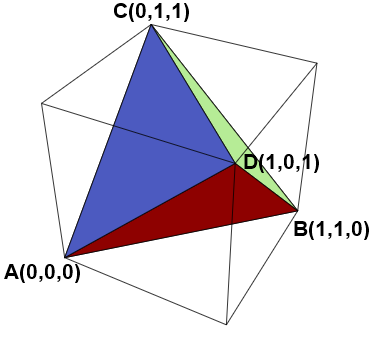### Compute the volume of a tetrahedron in SAS Since the volume formula includes vectors and vector operations, it is natural to use the SAS/IML language to compute the volume. The following functions implement the definition of the vector cross-product. To test the function, the program computes the volume of the largest tetrahedron that can be imbedded in the unit cube. One such tetrahedron is shown to the right. The tetrahedron has vertices A(0,0,0), B(1,1,0), C(0,1,1), and D(1,0,1). The volume of this tetrahedron is 1/3, as shown by the following program. /* volume of tetrahedron in 3D */ proc iml; /* vector cross product (3D vectors only) https://blogs.sas.com/content/iml/2013/05/20/matri-multiplication.html */ start CrossProd(u, v); i231 = {2 3 1}; i312 = {3 1 2}; return( u[i231]#v[i312] - v[i231]#u[i312] ); finish; start VolumeTetra(A,B,C,D); z = CrossProd(B-A, C-A); /* z = AB x AC */ w = colvec(D - A); /* w = AD */ volume = abs(z*w) / 6; /* V = 1/6 | z*w | */ return( volume ); finish; /* test the formula: compute the volume of the largest tetrahedron inside the unit cube */ A = {0 0 0}; B = {1 1 0}; C = {0 1 1}; D = {1 0 1}; maxVol = VolumeTetra(A,B,C,D); print maxVol;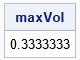### A Monte Carlo simulation of random tetrahedron It is not hard to write a SAS/IML program that generates four random vertices for a tetrahedron inside the unit cube in 3-D. To generate the four vertices, you can generate 12 uniform variates independently in the interval [0,1]. Assign the first three numbers to be the (x,y,z) Euclidean coordinates of the first vertex, the next three numbers to be the coordinates of the second vertex, and so forth. From the vertices, you can call the VolumeTetra function to produce the volume. The following program generates 100,000 random tetrahedra and computes their volumes. The program then calls the MEAN function computes the average volume, which is the Monte Carlo estimate of the expected value. call randseed(1234); N = 1E5; X = randfun(N//12, "Uniform"); /* Z x 12 matrix of uniform variates */ Vol = j(N, 1, .); /* allocate array for volumes */ do i = 1 to N; A = X[i, 1:3]; B = X[i, 4:6]; /* form four vertices from the 12 random numbers */ C = X[i, 7:9]; D = X[i, 10:12]; Vol[i] = VolumeTetra(A,B,C,D); end; avgVol = mean(Vol); /* Monte Carlo estimate of mean */ expectedValue = 3977/216000 - constant('pi')**2 / 2160; /* Zinani's exact result for E[Volume] */ print avgVol expectedValue (expectedValue-avgVol)[L="Diff"];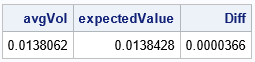The output shows that the Monte Carlo estimate is very close to the expected value. This confirms Zinani's result: the average volume of a random tetrahedron is smaller than you might think. ### Visualize the distribution of volumes The expected value for the distribution of tetrahedral volumes is small. Since we know that at least one tetrahedron has a relatively large value (1/3), we might conjecture that most of the tetrahedra have very small volumes and only a few have larger volumes. A way to test that conjecture is to visualize the distribution by drawing a histogram of the volumes for the 100,000 random tetrahedra. The following SAS/IML statements write the expected value to a macro variable and write the volumes of the random tetrahedra to a data set. PROC UNIVARIATE then computes descriptive statistics for the volumes and plots a histogram of the distribution of volumes: /* E[Volume] = 3977 / 216000 - pi**2 / 2160 = 0.01384277574... */ call symputx("EV",expectedValue); /* write E[Volume] to a macro variable */ create Vol var "Vol"; append; close; /* write volumes to a data set */ QUIT; title "Volume of a Random Tetrahedron"; proc univariate data=Vol; histogram Vol / endpoints href=&EV odstitle=title; run;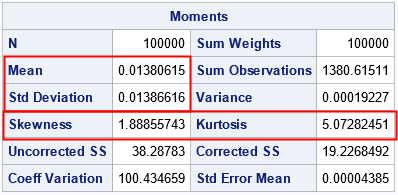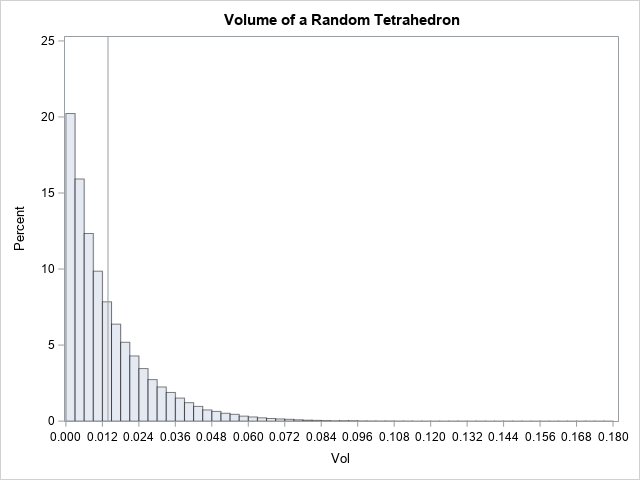The graph indicates that a random tetrahedron is likely to have a volume that is near 0. The vertical line is the expected value of the distribution. Notice that only a small proportion of the tetrahedra have volumes that exceed 0.1. In this collection of 100,000 random tetrahedra, the largest volume was 0.1777, which is about 50% of the volume of the largest possible tetrahedron. The distribution has a long but thin tail. If you look carefully at the table of descriptive statistics (the "Moments" table), you will see that the mean and the standard deviation are nearly the same. This property is characteristic of an exponential distribution. An exponential distribution has a skewness of 2 and an excess kurtosis of 6. However, the sample skewness and sample kurtosis are biased statistics, and the estimates shown here (1.8 and 5.1, respectively) are typical for a large sample from an exponential distribution. This suggests that the tetrahedral volume is distributed as V ~ Exp(λ=E[Volume]). Let's use PROC UNIVARIATE again and overlay the density of the Exp(λ=0.0139) distribution. Furthermore, you can use a Q-Q plot to visualize how well the exponential model fits the simulated volumes. proc univariate data=Vol; histogram Vol / exponential(theta=0 sigma=&EV) endpoints; qqplot Vol / exponential(theta=0 sigma=&EV); run;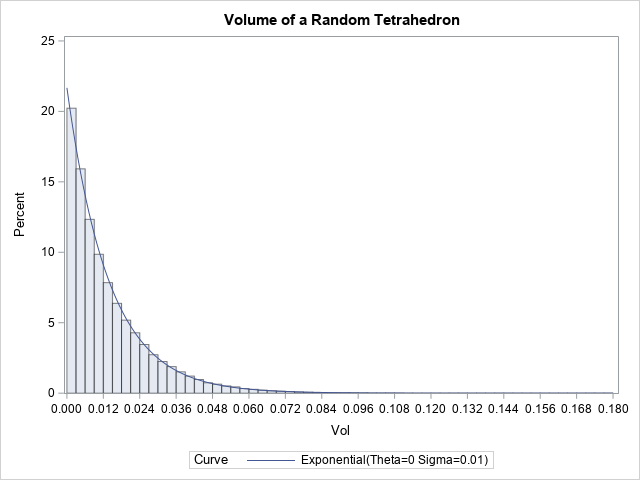The fit is excellent! Not only does the density curve overlay perfectly on the histogram, but the quantiles of the data (not shown) are in excellent agreement with the expected quantiles of the exponential distribution. If you look at the Q-Q plot, you again see excellent agreement between the data and the exponential model. The only shortcoming is that the simulated volumes have fewer extreme values than predicted by the model.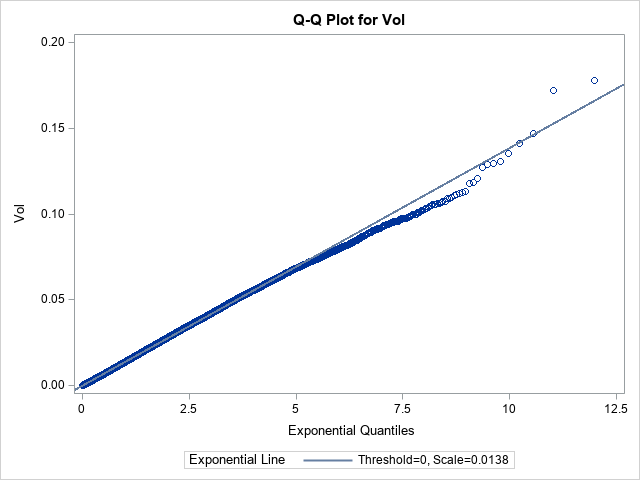The model enables you to compute the probability of certain events. For example, the probability that a random tetrahedron has volume greater than 0.1 is 1-cdf("Expo", 0.1, &EV), which is 0.00073. ### Summary A result by Zinani (2003) gives the expected volume of a tetrahedron whose vertices are chosen uniformly at random in the unit cube. Zinani's result is E[Volume] = 0.0138427757.... Although this number seems surprisingly small, the expected value is confirmed by using a Monte Carlo simulation in SAS. The simulation suggests that the volumes are exponentially distributed. For an alternative mathematical proof of the result, see Philip, J. (2007) "The Expected Volume of a Random Tetrahedron in a Cube", which is free to view and is very well written. The post The expected volume of a random tetrahedron in a cube appeared first on The DO Loop. A common question on SAS discussion forums is how to use SAS to generate random ID values. The use case is to generate a set of random strings to assign to patients in a clinical study. If you assign each patient a unique ID and delete the patients' names, you can preserve the patients' privacy during data analysis. A common requirement is that the strings be unique. This presents a potential problem. In a set of random values, duplicate values are expected. Think about rolling a six-sided die several times: you would expect to see a duplicate value appear after a few rolls. Even for a larger set of possible values, duplicates arise surprisingly often. For example, if a room contains 23 people, the Birthday Paradox shows that it is likely that two people in the room have the same birthday! If you generate N random numbers independently, you will likely encounter duplicates. There are several ways to use SAS to achieve both randomness and unique values. Perhaps the easiest way is to start with a long list of unique values, then randomly select N of these values. You can do the selection in either of two ways: 1. Generate a random permutation of these values. Assign the first N permuted values to the subjects. 2. Use sampling without replacement to select N values from the long list. A third way to obtain random strings without duplicates is to use a SAS/IML program. This article shows how to use SAS to generate random four-character strings. You can use the strings as IDs for subjects in a study that requires hiding the identity of the subjects. The article also discusses an important consideration: some four-character strings are uncomplimentary or obscene. I show how to remove vulgar strings from the list of four-character strings so that no patient is assigned an ID such as 'SCUM' or 'SPAZ'. ### A mapping between strings and integers I have previously shown that you can associate each string of English characters with a positive integer. For most applications, it suffices to consider four-digit strings because there are 264 = 456,976 strings that contains four English characters from the set {A, B, C, ..., Z}. Thus, I will use four-character strings in this article. (If you use five-character strings, you can assign up to 11,881,376 unique IDs.) The following SAS DATA step outputs the integers 0 through 264-1. For each integer, the program also creates a four-character string. Small integers are padded with "leading zeros," so that the integers 0, 1, 2, ..., 456975, are associated with the base 26 strings AAAA, AAAB, AAAC, ..., ZZZZ. See the previous article to understand how the program works. /* Step 1: Generate all integers in the range [0, 26^k -1] for k=4. See https://blogs.sas.com/content/iml/2022/09/14/base-26-integer-string.html Thanks to KSharp for the suggestion to use the RANK and BYTE functions: rank('A') = 65 in ASCII order byte(rank('A') + j) is the ASCII character for the j_th capital letter, j=0,1,...,25 */ %let nChar = 4; /* number of characters in base 26 string */ data AllIDs; array c[0:%eval(&nChar-1)] _temporary_ ; /* integer coefficients c, c, ... */ length ID$&nChar; /* string for base b */ b = 26; /* base b = 26 */ offset = rank('A'); /* = 65 for ASCII order */ do nID = 0 to b**&nChar - 1; /* compute the coefficients that represent nID in Base b */ y = nID; do k = 0 to &nChar - 1; c[k] = mod(y, b); /* remainder when r is divided by b */ y = floor(y / b); /* whole part of division */ substr(ID,&nChar-k,1) = byte(offset+c[k]); /* represent coefficients as string */ end; /* Some strings are vulgar. Exclude strings on the denylist. */ if ID not in ( 'CRAP','DAMN','DUMB','HELL','PUKE','SCUM','SLUT','SPAZ' /* add F**K, S**T, etc */ ) then output; end; drop b offset y k; run;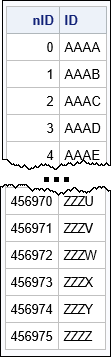The table shows the first few and the last few observations in the data set. The ID column contains all four-character strings of English letters, except for words on a denylist. The list of objectionable words can be quite long, so I included only a few words as an example. I left out the F-bomb, other vulgar terms, and racist slurs. The appendix contains a more complete denylist of four-letter words.

### Random permutation of the complete list

The first step created a list of unique four-character ID values. The second step is to randomly select N elements from this list, where N is the number of subjects in your study that need a random ID. This section shows how to perform random selection by permuting the entire list and selecting the first N rows of the permuted data.

The following DATA step generates a random uniform number for each ID value. A call to PROC SORT then sorts the data by the random values. The resulting data set, RANDPERM, has randomly ordered ID values.

/* Option 1: Generate a random permutation of the IDs */ data RandPerm; set AllIDs; call streaminit(12345); _u = rand("uniform"); run; /* sort by the random variate */ proc sort data=RandPerm; by _u; run; /* proc print data=RandPerm(obs=10) noobs; var nID ID; run; */

You can use PROC PRINT to see that the ID values are randomly ordered. Because we started with a list of unique values, the permuted IDs are also unique.

You can now merge the first N random IDs with your data. For example, suppose I want to assign an ID to each student in the Sashelp.Class data set. The following program counts how many subjects (N) are in the data, and merges the first N random IDs with the data:

/* Count how many rows (N) are in the input data set */ %let dsName = sashelp.class; proc sql noprint; select count(*) into :N from &DSName; quit;   data All; merge &DSName RandPerm(keep=ID obs=&N); run;   proc print data=All(obs=8) noobs label; var ID Name _NUMERIC_; run;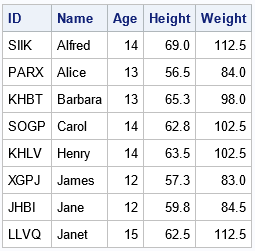The output shows that each student is assigned a random four-character ID.

### Use PROC SURVEYSELECT to select IDs

The previous section uses only Base SAS routines: The DATA step and PROC SQL. An alternative approach is to extract N random IDs by using PROC SURVEYSELECT in SAS/STAT software. In this approach, you do not need to generate random numbers and manually sort the IDs. Instead, you extract the random values by using PROC SURVEYSELECT. As of SAS 9.4 M5, the SURVEYSELECT procedure supports the OUTRANDOM option, which causes the selected items in a simple random sample to be randomly permuted after they are selected. Thus, an easier way to assign random IDs is to count the number of subjects, randomly select that many ID values from the (unsorted) set of all IDs, and then merge the results:

/* Option 2: Extract random IDs and merge */ %let dsName = sashelp.class; proc sql noprint; select count(*) into :N from &DSName; quit;   proc surveyselect data=AllIDs out=RandPerm noprint seed=54321 method=srs /* sample w/o replacement */ OUTRANDOM /* SAS 9.4M5 supports the OUTRANDOM option */ sampsize=&N; /* number of observations in sample */ run;   data All; merge &DSName RandPerm(keep=ID); run;   proc print data=All(obs=8) noobs label; var ID Name _NUMERIC_; run;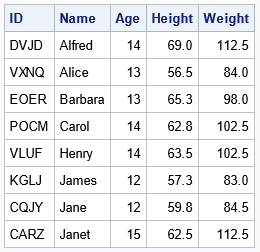The table shows the ID values that were assigned to the first few subjects.

### A SAS/IML method

Both previous methods assign random strings of letters to subjects. However, I want to mention a third alternative because it is so compact. You can write a SAS/IML program that performs the following steps:

• Create a vector that contains the 26 English letters.
• Use the EXPANDGRID function to create a matrix that contains all four-character combinations of letters. Concatenate those letters into strings.
• Optionally, remove objectional strings. You can use the ELEMENT function to identify the location of strings on a denylist.
• Use the SAMPLE function to generate a random sample (without replacement).

As usual, the SAS/IML version is very compact. It requires about a dozen lines of code to generate the IDs and write them to a data set for merging with the subject data:

/* Generate N random 4-character strings (remove words on denylist) */ proc iml; letters = 'A':'Z'; /* all English letters */ L4 = expandgrid(letters, letters, letters, letters); /* 26^4 combinations */ strings = rowcat(L4); /* concatenate into strings */ free L4; /* done with matrix; delete */   deny = {'CRAP','DAMN','DUMB','HELL','PUKE','SCUM','SLUT','SPAZ'}; /* add F**K, S**T, etc*/ idx = loc( ^element(strings, deny) ); /* indices of strings NOT on denylist */ ALLID = strings[idx];   call randseed(1234); ID = sample(strings, &N, "WOR"); /* random sample without replacement */ create RandPerm var "ID"; append; close; /* write IDs to data set */ QUIT;   /* merge data and random ID values */ data All; merge &DSName RandPerm; run;   proc print data=All(obs=8) noobs label; var ID Name _NUMERIC_; run;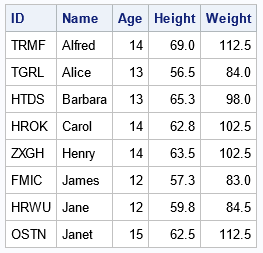### Summary

This article shows how to assign a random string value to subjects in an experiment. The best way to do this is to start with a set of unique strings. Make sure there are no objectionable words in the set! Then you can extract a random set of N strings by permuting the set or by using PROC SURVEYSELECT to perform simple random sampling (SRS). In the SAS/IML language, you can generate all strings and extract a random subset by using only a dozen lines of code.

In a DATA step, I used base 26 to generate the list of all four-character strings. By changing the definition of the nChar macro variable, this program also works for character strings of other lengths. If you set the macro to the value k, the program will generate 26k strings of length k.

Of the three methods, the first (DATA step and PROC SORT) is the simplest. It can also easily handle new subjects who are added to the study after the initial assignment of IDs. You can simply assign the next unused random IDs to the new subjects.

### Appendix: A denylist of four-letter words

This appendix shows a list of objectionable words. You do not want to assign a patient ID that is obscene, profane, insulting, racist, sexist, or crude. I used several web pages to compile a list of four-letter words that some people might find objectionable. You can use an IF-THEN statement to block the following list four-letter English words:

/* some words on this list are from https://www.wired.com/2016/09/science-swear-words-warning-nsfw-af/ https://en.everybodywiki.com/List_of_profane_words */ if ID not in ( 'ANAL','ANUS','ARSE','BOOB','BUNG','BUTT','CLIT','COCK','COON','CRAP', 'CUMS','CUNT','DAMN','DICK','DONG','DUMB','DUMP','DYKE','FAGS','FUCK', 'GOOK','HEBE','HELL','HOMO','JEEZ','JERK','JISM','JIZZ','JUGS','KIKE', 'KNOB','KUNT','MICK','MILF','MOFO','MONG','MUFF','NADS','PAKI','PISS', 'POON','POOP','PORN','PUBE','PUKE','PUSS','PUTO','QUIM','SCUM','SHAG', 'SHAT','SHIT','SLAG','SLUT','SMEG','SPAZ','SPIC','SUCK','TARD','THOT', 'TOSS','TURD','TWIT','TWAT','WANK','WANG' ) then output;

The post Generate random ID values for subjects in SAS appeared first on The DO Loop.

When I was writing Simulating Data with SAS (Wicklin, 2013), I read a lot of introductory textbooks about Monte Carlo simulation. One of my favorites is Sheldon Ross's book Simulation. (I read the 4th Edition (2006); the 5th Edition was published in 2013.) I love that the book brings together many examples of short, easy-to-understand, applications of using Monte Carlo methods. Over the years, I have blogged about many of these applications, such as estimating π or e by using simulation in SAS.

These examples use random uniform variates to estimate a probability or an area, so they are understandable by anyone who is getting started with Monte Carlo simulation. This article compiles these elementary examples in one location. Each section briefly describes the problem and provides a link to an article that shows how to solve the problem in SAS.

### Estimate π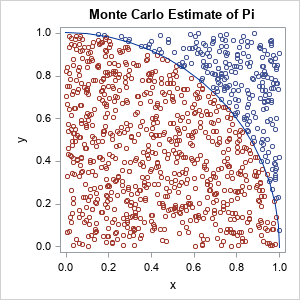There are multiple ways to formulate the problem of estimating π ≈ 3.14159.... The most famous one is called the "area method." You generate a large number of points uniformly at random in the unit square and count how many are also in the quarter circle Q = {(x,y) | x2 + y2 < 1, x ≥ 0, y ≥ 0}. The graph to the right illustrates this method. The area of the quarter-circle is estimated by the ratio NC / NS, where NC is the number of points inside the quarter circle and NS is the number of points inside the unit square. Because we know that the quarter-circle has area π / 4, an estimate for π is π ≈ 4 NC / NS. Read more about estimating π as an area.

### Estimate the area of a planar figure

The Monte Carlo method can be used to estimate the area of any bounded planar figure. You merely need a bounding box and a way to assess whether a random point is inside the figure. Read more about estimating areas by using Monte Carlo techniques.

### Buffon's needle problem

One of the many methods for estimating π was introduced by a minor French nobleman, G.-L. Leclerc, the Comte de Buffon (the Count of Buffon). According to legend, the Count dropped a stack of needles on a hardwood floor that had strips of wood aligned in parallel. He noticed that some needles crossed the cracks between boards whereas others dd not. He then formulated one of the first problems in geometric probability: What is the probability that a random needle crosses a line? The general answer depends on the relative width of the lines and the lengths of needles, but if the length of the needle equals the width between boards, the probability is 2 / π. By simulating this experiment, you can estimate π. Read more about Monte Carlo simulation of Buffon's needle.

This problem is also connected to an early example of academic dishonesty. In 1901, Mario Lazzarini claims to have tossed a needle 3,408 times and obtained an estimate of π that is accurate to six decimal places. He almost surely lied about doing the experiment, but his fraud reminds us to critically review claims that seem too good to be true. It is easy to use a simulation to lie and deceive.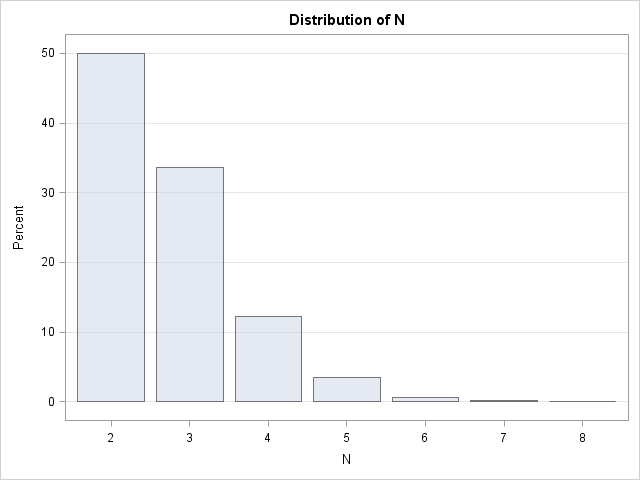### Estimate e

If a computation involves a circle, it is not surprising if π appears in the answer. However, it is less common to see the base of the natural logarithm, e ≈ 2.71828..., appear in a computation. Nevertheless, e is the answer to a very simple problem. Let N be the minimum number of draws from a uniform distribution such that the sum of the variates exceeds 1. What is the expected value of N? We know the answer is greater than 1 and intuition suggests that the answer is greater than 2. With some math and geometry, you can determine that the expected number of variates is e. A Monte Carlo simulation is easy to program by using the SAS DATA step in about 10 lines of code. Read more about how to estimate e by using a probability.

### Estimate a one-dimensional integral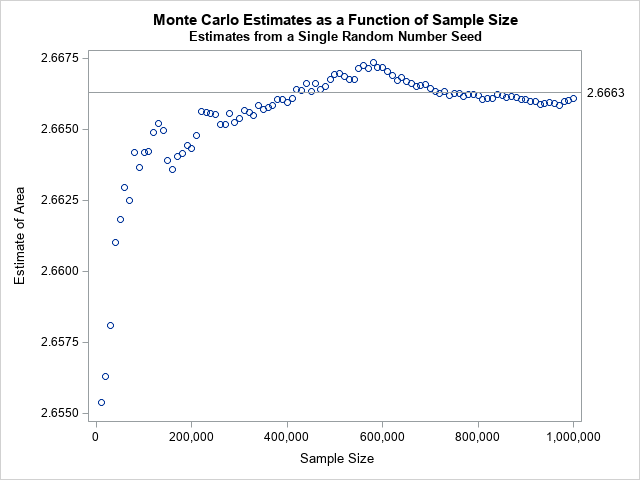Using the Monte Carlo method to estimate an integral is an eye-opening experience. To estimate the integral of a continuous function g on the interval (a,b), you can generate a uniform random sample in (a,b), evaluate g on each point in the sample, and take the arithmetic mean of those values. In symbols,
$\int_{a}^{b} g(x) \,dx \approx (b - a) \cdot \frac{1}{n} \sum\nolimits_{i = 1}^{n} g(x_{i})$
where the $x_i$ are independent random uniform variates on (a,b). Read more about Monte Carlo estimates of a one-dimensional integral.

### Estimate a higher-dimensional integral

There are many numerical methods for solving one-dimensional integrals. There are few methods for solving high-dimensional integrals. For a rectangular domain, the Monte Carlo method is amazingly simple to implement. For a non-rectangular domain, the method is more complex but still simpler than many competing methods that are taught in multivariate calculus courses. Read more about Monte Carlo estimates of a two-dimensional integral.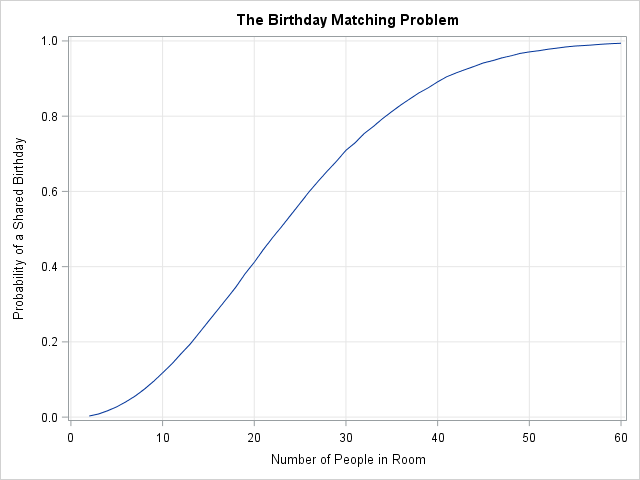### The birthday-matching problem

The famous birthday-matching problem (sometimes called the birthday paradox) states that in a room that contains N people, the probability that two people share a birthday is much higher than intuition suggests. In a room of N=23 people, the probability that two people share a birthday is more than 56%. For N=30, the probability is more than 70%. Many people do not believe the result until they write a simulation that demonstrates it is true. Read more about the birthday-matching problem.

### The Monty Hall problem

Monty Hall was a game-show host. The Monty Hall problem is a question about conditional probability whose answer is counter-intuitive. Suppose you're on a game show, and you're given the choice of three doors: Behind one door is a car; behind the others, goats. You pick a door, say No. 1, and the host [Monty Hall], who knows what's behind the doors, opens another door, say No. 3, which has a goat. He then says to you, "Do you want to pick door No. 2?" Is it to your advantage to switch your choice? (A goat was a funny way to show that the contestant did not win a prize.)

Many people argue that either you picked the door that contains the prize, or you didn't, so it doesn't make any sense to switch. However, it turns out that if you switch, the probability of winning is 2/3, whereas if you do not switch the probability of winning is 1/3. So, it pays to change your mind! Read more about the Monty-Hall problem.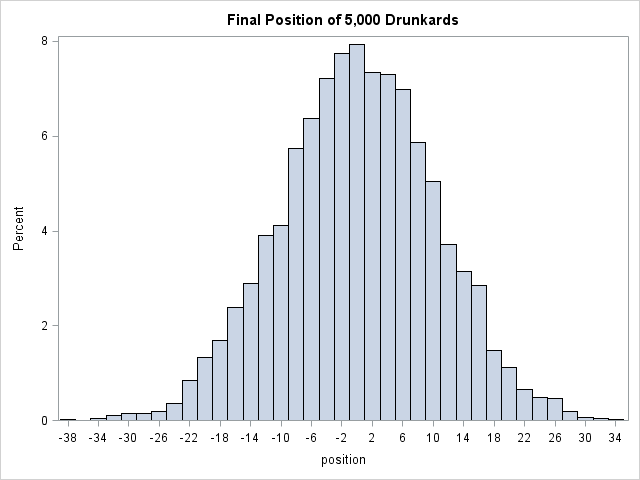### The drunkard's walk

The drunkard's walk is one kind of random walk. The problem asks for the probability that a drunkard (who takes a unit step in a random coordinate direction) returns to his starting location after N steps. However, the same computation can be used to estimate the probability that he ends up at any other location on a unit grid. You can read about how to simulate the drunkard's walk in one dimension, or generalize the problem to simulate a drunkard's walk in two dimensions.

### Summary

This article presents several classic examples that use Monte Carlo simulation to estimate an area or a probability. The examples are easy to follow because they simulate from uniform distributions and do not require any advanced knowledge of probability or statistics. As such, these problems are ideal for someone who wants to begin learning how to perform Monte Carlo simulations in SAS. This article collects these examples in one convenient location.

The post Introductory examples of Monte Carlo simulation in SAS appeared first on The DO Loop.

I've previously shown how to use Monte Carlo simulation to estimate probabilities and areas. I illustrated the Monte Carlo method by estimating π ≈ 3.14159... by generating points uniformly at random in a unit square and computing the proportion of those points that were inside the unit circle. The previous article used the SAS/IML language to estimate the area. However, you can also perform a Monte Carlo computation by using the SAS DATA step. Furthermore, the method is not restricted to simple geometric shapes like circles. You can use the Monte Carlo technique to estimate the area of any bounded planar figure. You merely need a bounding box and a way to assess whether a random point is inside the figure. This article uses Monte Carlo simulation to estimate the area of a polar rose with five petals.

### The polar rose

You can read about how to draw a polar rose in SAS. The simplest form of the polar rose is given by the polar equation r = cos(k θ), where k is an integer, r is the polar radius, and θ is the polar angle (0 ≤ θ ≤ 2 π).

The following DATA step computes the coordinates of the polar rose for k=5. The subsequent call to PROC SGPLOT graphs the curve.

%let k = 5; /* for odd k, the rose has k petals */ /* draw the curve r=cos(k * theta) in polar coordinates: https://blogs.sas.com/content/iml/2015/12/16/polar-rose.html */ data Rose; do theta = 0 to 2*constant("pi") by 0.01; r = cos(&k * theta); /* rose */ x = r*cos(theta); /* convert to Euclidean coordinates */ y = r*sin(theta); output; end; keep x y; run;   title "Polar Rose: r = cos(&k theta)"; ods graphics / width=400px height=400px; proc sgplot data=Rose aspect=1; refline 0 / axis=x; refline 0 / axis=y; series x=x y=y; xaxis min=-1 max=1; yaxis min=-1 max=1; run;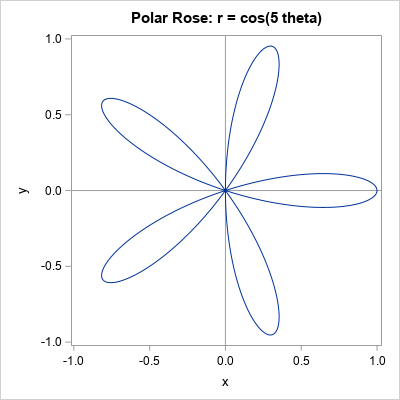The remainder of this article uses Monte Carlo simulation to estimate the area of the five-petaled rose. The answer is well-known and is often proved in a calculus class. When k is odd, the area of the rose generated by r = cos(k θ) is A = π / 4 ≈ 0.7854.

### Monte Carlo estimates of area

To estimate the area of a geometric figure by using Monte Carlo simulation, do the following:

1. Generate N points uniformly in a bounding rectangle.
2. For each point, compute whether the point is inside the figure. This enables you to form the proportion, p, of points that are inside the figure.
3. Estimate the area of the figure as p AR, where AR is the area of the bounding rectangle.
4. Optionally, you can construct a confidence interval for the area. A confidence interval for the binomial proportion is p ± zα sqrt(p(1-p) / N), where zα is the 1-α/2 quantile of the normal distribution. If you multiply the interval by AR, you get a confidence interval for the area.

The Monte Carlo method produces a simulation-based estimate. The answer depends on the random number stream, so you obtain AN estimate rather than THE estimate. In this article, the rose will be bounded by using the rectangle [-1, 1] x [-1, 1], which has area AR = 4.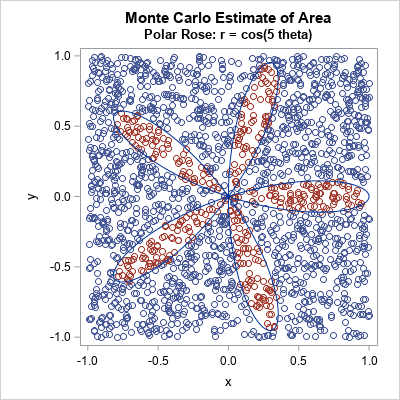The plot to the right shows the idea of a Monte Carlo estimate. The plot shows 3,000 points from the uniform distribution on the rectangle [-1, 1] x [-1, 1]. There are 600 points, shown in red, that are inside one of the petals of the polar rose. Because 600 is 20% of the total number of points, we estimate that the rose occupies 20% of the area of the square. Thus, with N=3000, an estimate of the area of the rose is 0.2*AR = 0.8, which is somewhat close to π / 4 ≈ 0.7854.

Statistical theory suggests that the accuracy of the proportion estimate should be proportional to 1/sqrt(N). This statement has two implications:

1. If you want to double the accuracy of an estimate, you must increase the number of simulated points fourfold.
2. Loosely speaking, if you use one million points (N=1E6), you can expect your estimate of the proportion to be roughly 1/sqrt(N) = 1E-3 units from the true proportion. A later section makes this statement more precise.

### A Monte Carlo implementation in the SAS DATA step

Let's increase the number of simulated points to improve the accuracy of the estimates. We will use one million (1E6) random points in the bounding rectangle.

There are two primary ways to obtain the Monte Carlo estimate. The first way is to generate a data set that has N random points and contains a binary indicator variable that specifies whether each point is inside the figure. You can then use PROC FREQ to obtain an estimate of the binomial proportion and a confidence interval for the proportion, as follows:

%let N = 1E6; /* generate N random points in bounding rectangle */ data Area; /* define rectangle on which to uniformly sample */ a = -1; b = 1; /* x in (a,b) */ c = -1; d = 1; /* y in (a,b) */ AreaRect = (b-a)*(d-c); /* area of bounding rectangle */ call streaminit(12345); do i = 1 to &N; x = rand("Uniform", a, b); y = rand("Uniform", c, d); /* Add code HERE to determine if (x,y) is inside the region of interest */ theta = atan2(y, x); /* notice order */ rxy = sqrt(x**2 + y**2); /* observed value of r */ isInside = (rxy < cos(&k * theta)); /* inside the rose */ output; end; keep x y isInside; run;   /* isInside is binary, so can compute binomial CI for estimate */ proc freq data=Area; tables IsInside / binomial(level='1' CL=wald); /* Optional: Use BINOMIAL option for CL */ ods select Binomial BinomialCLs; run;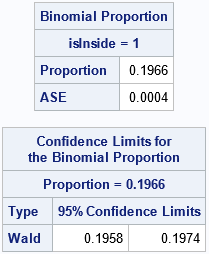The proportion of points inside the figure is 0.1966. A 95% confidence interval for the proportion is [0.1958, 0.1974]. To transform these values into areas, you need to multiply them by the area of the bounding rectangle, which is 4. Thus, an estimate for the area of the rose is 0.7864, and a confidence interval is [0.7832, 0.7896]. This estimate is within 0.001 units of the true area, which is π/4 ≈ 0.7854. If you change the random number seed, you will get a different estimate.

### Manual computation of binomial estimates

If you are comfortable with programming the binomial proportion yourself, you can perform the entire computation inside a DATA step without using PROC FREQ. The advantage of this method is that you do not generate a data set that has N observations. Rather, you can count the number of random points that are inside the rose, and merely output an estimate of that area and a confidence interval. By using this method, you can simulate millions or billions of random points without writing a large data set. The following DATA step demonstrates this technique:

%let N = 1E6; data MCArea; /* define rectangle on which to uniformly sample */ a = -1; b = 1; /* x in (a,b) */ c = -1; d = 1; /* y in (a,b) */ AreaRect = (b-a)*(d-c); /* area of bounding rectangle */ call streaminit(12345); do i = 1 to &N; x = rand("Uniform", a, b); y = rand("Uniform", c, d); /* Add code HERE to determine if (x,y) is inside the region of interest */ theta = atan2(y, x); /* notice order */ rxy = sqrt(x**2 + y**2); /* observed value of r */ isInside = (rxy < cos(&k * theta)); /* inside the rose */ /* we now have binary variable isInside */ sumInside + isInside; /* count the number of points inside */ end;   /* simulation complete; output only the results */ p = sumInside / &N; /* proportion of points inside region */ AreaEst = p * AreaRect; /* estimate of area of the figure */ /* Optional: Since p is a binomial proportion, you can get a confidence interval */ alpha = 0.05; zAlpha = quantile("Normal", 1-alpha/2); /* = 1.96 when alpha=0.05 */ SE = zAlpha*sqrt(p*(1-p)/&N); LCL = (p - SE)*AreaRect; UCL = (p + SE)*AreaRect; /* Optional: if you know the true area, you can compare it with MC estimate */ ExactArea = constant('pi') / 4; Difference = ExactArea - AreaEst; keep AreaRect p AreaEst LCL UCL ExactArea Difference; label AreaRect="Area of Rectangle" p = "Proportion Inside" AreaEst="MC Area Estimate" ExactArea="Exact Area" LCL="95% Lower Limit" UCL="95% Upper Limit"; run;   proc print data=MCArea noobs label; format Difference 8.5; run;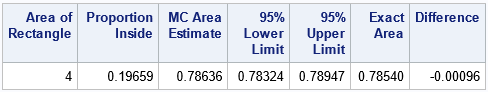Notice that this DATA step outputs only one observation, whereas the previous DATA step created N observations, which were later read into PROC FREQ to estimate the proportion. Also, this second program multiplies the proportion and the confidence interval by the area of the bounding rectangle. Thus, you get the estimates for the area of the figure, not merely the estimates for the proportion of points inside the figure. The two answers are the same because both programs use the same set of random points.

Regarding the accuracy of the computation, notice that the confidence interval for the proportion is based on the standard error computation, which is zα sqrt(p*(1-p)/N). This quantity is largest when p=1/2 and zα = 1.96 for α = 0.05. Thus, for any p, the standard error is less than 1/sqrt(N). This is what I meant earlier when I claimed that the estimate of the proportion is likely to be within 1/sqrt(N) units from the true proportion. Of course, your estimates for the area might not be that small if the area of the bounding rectangle is large. The estimate of the area is likely to be AR/sqrt(N) units from the true area. But by choosing N large enough, you can make the error as small as you like.

### Summary

This article shows how to use the SAS DATA step to perform a Monte Carlo approximation for the area of a bounded planar figure, the five-petaled polar rose. The key to the computation is being able to assess whether a random point is inside the figure of interest. If you can do that, you can estimate the area by using the proportion of random points inside the figure times the area of the bounding rectangle.

The article shows two implementations. The first writes the binary indicator variable to a SAS data set and uses PROC FREQ to compute the proportion estimate. To estimate the are, multiply the proportion by the area of the bounding rectangle. The second implementation performs all computations inside the DATA step and outputs only the estimates of the proportion and the area.

The post Monte Carlo estimates of area appeared first on The DO Loop.

I've previously shown how to use Monte Carlo simulation to estimate probabilities and areas. I illustrated the Monte Carlo method by estimating π ≈ 3.14159... by generating points uniformly at random in a unit square and computing the proportion of those points that were inside the unit circle. The previous article used the SAS/IML language to estimate the area. However, you can also perform a Monte Carlo computation by using the SAS DATA step. Furthermore, the method is not restricted to simple geometric shapes like circles. You can use the Monte Carlo technique to estimate the area of any bounded planar figure. You merely need a bounding box and a way to assess whether a random point is inside the figure. This article uses Monte Carlo simulation to estimate the area of a polar rose with five petals.

### The polar rose

You can read about how to draw a polar rose in SAS. The simplest form of the polar rose is given by the polar equation r = cos(k θ), where k is an integer, r is the polar radius, and θ is the polar angle (0 ≤ θ ≤ 2 π).

The following DATA step computes the coordinates of the polar rose for k=5. The subsequent call to PROC SGPLOT graphs the curve.

%let k = 5; /* for odd k, the rose has k petals */ /* draw the curve r=cos(k * theta) in polar coordinates: https://blogs.sas.com/content/iml/2015/12/16/polar-rose.html */ data Rose; do theta = 0 to 2*constant("pi") by 0.01; r = cos(&k * theta); /* rose */ x = r*cos(theta); /* convert to Euclidean coordinates */ y = r*sin(theta); output; end; keep x y; run;   title "Polar Rose: r = cos(&k theta)"; ods graphics / width=400px height=400px; proc sgplot data=Rose aspect=1; refline 0 / axis=x; refline 0 / axis=y; series x=x y=y; xaxis min=-1 max=1; yaxis min=-1 max=1; run;The remainder of this article uses Monte Carlo simulation to estimate the area of the five-petaled rose. The answer is well-known and is often proved in a calculus class. When k is odd, the area of the rose generated by r = cos(k θ) is A = π / 4 ≈ 0.7854.

### Monte Carlo estimates of area

To estimate the area of a geometric figure by using Monte Carlo simulation, do the following:

1. Generate N points uniformly in a bounding rectangle.
2. For each point, compute whether the point is inside the figure. This enables you to form the proportion, p, of points that are inside the figure.
3. Estimate the area of the figure as p AR, where AR is the area of the bounding rectangle.
4. Optionally, you can construct a confidence interval for the area. A confidence interval for the binomial proportion is p ± zα sqrt(p(1-p) / N), where zα is the 1-α/2 quantile of the normal distribution. If you multiply the interval by AR, you get a confidence interval for the area.

The Monte Carlo method produces a simulation-based estimate. The answer depends on the random number stream, so you obtain AN estimate rather than THE estimate. In this article, the rose will be bounded by using the rectangle [-1, 1] x [-1, 1], which has area AR = 4.The plot to the right shows the idea of a Monte Carlo estimate. The plot shows 3,000 points from the uniform distribution on the rectangle [-1, 1] x [-1, 1]. There are 600 points, shown in red, that are inside one of the petals of the polar rose. Because 600 is 20% of the total number of points, we estimate that the rose occupies 20% of the area of the square. Thus, with N=3000, an estimate of the area of the rose is 0.2*AR = 0.8, which is somewhat close to π / 4 ≈ 0.7854.

Statistical theory suggests that the accuracy of the proportion estimate should be proportional to 1/sqrt(N). This statement has two implications:

1. If you want to double the accuracy of an estimate, you must increase the number of simulated points fourfold.
2. Loosely speaking, if you use one million points (N=1E6), you can expect your estimate of the proportion to be roughly 1/sqrt(N) = 1E-3 units from the true proportion. A later section makes this statement more precise.

### A Monte Carlo implementation in the SAS DATA step

Let's increase the number of simulated points to improve the accuracy of the estimates. We will use one million (1E6) random points in the bounding rectangle.

There are two primary ways to obtain the Monte Carlo estimate. The first way is to generate a data set that has N random points and contains a binary indicator variable that specifies whether each point is inside the figure. You can then use PROC FREQ to obtain an estimate of the binomial proportion and a confidence interval for the proportion, as follows:

%let N = 1E6; /* generate N random points in bounding rectangle */ data Area; /* define rectangle on which to uniformly sample */ a = -1; b = 1; /* x in (a,b) */ c = -1; d = 1; /* y in (a,b) */ AreaRect = (b-a)*(d-c); /* area of bounding rectangle */ call streaminit(12345); do i = 1 to &N; x = rand("Uniform", a, b); y = rand("Uniform", c, d); /* Add code HERE to determine if (x,y) is inside the region of interest */ theta = atan2(y, x); /* notice order */ rxy = sqrt(x**2 + y**2); /* observed value of r */ isInside = (rxy < cos(&k * theta)); /* inside the rose */ output; end; keep x y isInside; run;   /* isInside is binary, so can compute binomial CI for estimate */ proc freq data=Area; tables IsInside / binomial(level='1' CL=wald); /* Optional: Use BINOMIAL option for CL */ ods select Binomial BinomialCLs; run;The proportion of points inside the figure is 0.1966. A 95% confidence interval for the proportion is [0.1958, 0.1974]. To transform these values into areas, you need to multiply them by the area of the bounding rectangle, which is 4. Thus, an estimate for the area of the rose is 0.7864, and a confidence interval is [0.7832, 0.7896]. This estimate is within 0.001 units of the true area, which is π/4 ≈ 0.7854. If you change the random number seed, you will get a different estimate.

### Manual computation of binomial estimates

If you are comfortable with programming the binomial proportion yourself, you can perform the entire computation inside a DATA step without using PROC FREQ. The advantage of this method is that you do not generate a data set that has N observations. Rather, you can count the number of random points that are inside the rose, and merely output an estimate of that area and a confidence interval. By using this method, you can simulate millions or billions of random points without writing a large data set. The following DATA step demonstrates this technique:

%let N = 1E6; data MCArea; /* define rectangle on which to uniformly sample */ a = -1; b = 1; /* x in (a,b) */ c = -1; d = 1; /* y in (a,b) */ AreaRect = (b-a)*(d-c); /* area of bounding rectangle */ call streaminit(12345); do i = 1 to &N; x = rand("Uniform", a, b); y = rand("Uniform", c, d); /* Add code HERE to determine if (x,y) is inside the region of interest */ theta = atan2(y, x); /* notice order */ rxy = sqrt(x**2 + y**2); /* observed value of r */ isInside = (rxy < cos(&k * theta)); /* inside the rose */ /* we now have binary variable isInside */ sumInside + isInside; /* count the number of points inside */ end;   /* simulation complete; output only the results */ p = sumInside / &N; /* proportion of points inside region */ AreaEst = p * AreaRect; /* estimate of area of the figure */ /* Optional: Since p is a binomial proportion, you can get a confidence interval */ alpha = 0.05; zAlpha = quantile("Normal", 1-alpha/2); /* = 1.96 when alpha=0.05 */ SE = zAlpha*sqrt(p*(1-p)/&N); LCL = (p - SE)*AreaRect; UCL = (p + SE)*AreaRect; /* Optional: if you know the true area, you can compare it with MC estimate */ ExactArea = constant('pi') / 4; Difference = ExactArea - AreaEst; keep AreaRect p AreaEst LCL UCL ExactArea Difference; label AreaRect="Area of Rectangle" p = "Proportion Inside" AreaEst="MC Area Estimate" ExactArea="Exact Area" LCL="95% Lower Limit" UCL="95% Upper Limit"; run;   proc print data=MCArea noobs label; format Difference 8.5; run;`Notice that this DATA step outputs only one observation, whereas the previous DATA step created N observations, which were later read into PROC FREQ to estimate the proportion. Also, this second program multiplies the proportion and the confidence interval by the area of the bounding rectangle. Thus, you get the estimates for the area of the figure, not merely the estimates for the proportion of points inside the figure. The two answers are the same because both programs use the same set of random points.

Regarding the accuracy of the computation, notice that the confidence interval for the proportion is based on the standard error computation, which is zα sqrt(p*(1-p)/N). This quantity is largest when p=1/2 and zα = 1.96 for α = 0.05. Thus, for any p, the standard error is less than 1/sqrt(N). This is what I meant earlier when I claimed that the estimate of the proportion is likely to be within 1/sqrt(N) units from the true proportion. Of course, your estimates for the area might not be that small if the area of the bounding rectangle is large. The estimate of the area is likely to be AR/sqrt(N) units from the true area. But by choosing N large enough, you can make the error as small as you like.

### Summary

This article shows how to use the SAS DATA step to perform a Monte Carlo approximation for the area of a bounded planar figure, the five-petaled polar rose. The key to the computation is being able to assess whether a random point is inside the figure of interest. If you can do that, you can estimate the area by using the proportion of random points inside the figure times the area of the bounding rectangle.

The article shows two implementations. The first writes the binary indicator variable to a SAS data set and uses PROC FREQ to compute the proportion estimate. To estimate the are, multiply the proportion by the area of the bounding rectangle. The second implementation performs all computations inside the DATA step and outputs only the estimates of the proportion and the area.

The post Monte Carlo estimates of area appeared first on The DO Loop.